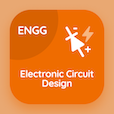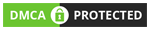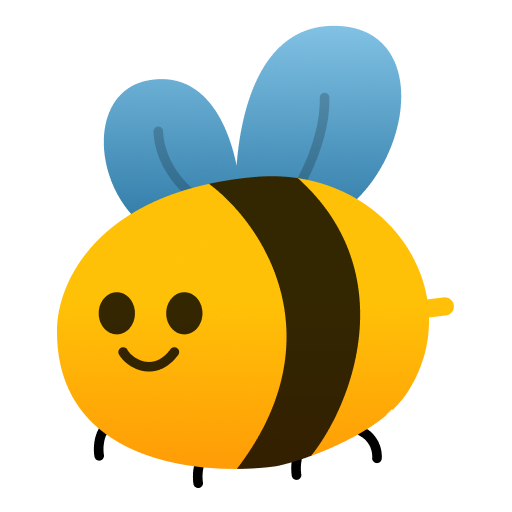Online CS Degree Courses

Android MCQs

Introduction to Computer Questions and Answers Multiple Choice Questions (MCQ Quiz), Android quiz answers PDF to learn android online course for android classes. for Statement Multiple Choice Questions and Answers (MCQs), Android quiz questions for software engineering online courses. Introduction to Computer Questions and Answers Book PDF: learning c language with interactive questions and answers, introduction to computer questions and answers, c programming practice exercises with answers test prep for online computer science and engineering.

"Evaluating the following code <code>for(i=0; i<7; ++i) {<br> for (j=0; j<i; ++j ) <br> printf ("%d\n", i*j )} </code>, the ratio of printf statement execution in first call is" MCQ PDF: introduction to computer questions and answers App APK with 7, 1, 0, and 6 choices for software engineering online courses. Learn introduction to computer questions and answers quiz questions for merit scholarship test and certificate programs for top computer science schools.

## MCQs on Introduction to Computer Questions and Answers Quiz

MCQ: Evaluating the following code `for(i=0; i<7; ++i) { for (j=0; j printf ("%d\n", i*j )} `, the ratio of printf statement execution in first call is

7
1
0
6

MCQ: By evaluating the following statements where x =3, y= 5 and z = 2:` x *= y+z; y /= 2*z+1; z += x; ` , the values of x,y and z are

x=21, y=1, z=23
x=1, y=21, z=23
x=1, y=23, z=21
x=21, y=23, z=1

MCQ: Evaluating the following code `for(i=0; i<7; ++i) { for (j=0; j printf ("%d\n", i*j )} `, the ratio of printf statement execution in second call is

7
1
0
6

MCQ: Evaluating the following code `for(i=0; i<7; ++i) { for (j=0; j printf ("%d\n", i*j )} `, the last value displayed is

30
21
20
12

MCQ: By evaluating the following statements where j = 5 and k = 2:` n=j - ++k; m= j-- + k--; p = k + j; ` , the values of n,m and p are

n=2, p=8, m=6
n=6, p=2, m=8
n=8, p=2, m=6
n=2, p=6, m=8

### More Topics from Android Book``` ```
```General Home Site Map Privacy Policy Affiliate Disclosure Contact Us Social Links Facebook Twitter YouTube Mobile Apps Android iOSAs an Amazon Associate I earn from qualifying purchases. MCQsLearn Web Portal Copyright © 2014-2022. All rights reserved.Try our FREE mobile apps for an amazing & interactive experience. Buy PREMIUM with trial period to see all analytics, complete history, answer keys to improve your premium insights. We assure you to enjoy PREMIUM! function checkAttempt(element, number) { var answer = document.getElementById("answer-" + number).innerHTML; var options = Array.from(document.getElementsByClassName("option-" + number)); if (answer == element.id.split("-")) { element.classList.add("correct-option"); options.forEach(function(option) { option.classList.add("disabled"); }); } else { element.classList.add("wrong-option"); options.forEach(function(option) { option.classList.add("disabled"); if (answer == option.id.split("-")) { \$("." + option.classList).delay(500).queue(function() { this.classList.add("correct-option"); }); } }); } } const validPage = window.location.href != "https://mcqslearn.com/apps/free-apple-android-apps-to-download.php"; function getCookie(cname) { let name = cname + "="; let decodedCookie = decodeURIComponent(document.cookie); let ca = decodedCookie.split(';'); for (let i = 0; i < ca.length; i++) { let c = ca[i]; while (c.charAt(0) == ' ') { c = c.substring(1); } if (c.indexOf(name) == 0) { return c.substring(name.length, c.length); } } return ""; } const _modal = document.getElementById("resetCourseModal"); const modal = new bootstrap.Modal(_modal); setTimeout(function() { if (!getCookie("initModal") && validPage) { modal.show(); const exp = new Date(); exp.setTime(exp.getTime() + (12 * 60 * 60 * 1000)); const utcExp = exp.toUTCString(); document.cookie = "initModal=true;expires=" + utcExp + ";path=/"; } }, 5000); document.body.addEventListener("mouseleave", function() { if (!getCookie("exitModal") && validPage) { modal.show(); const exp = new Date(); exp.setTime(exp.getTime() + (12 * 60 * 60 * 1000)); const utcExp = exp.toUTCString(); document.cookie = "exitModal=true;expires=" + utcExp + ";path=/"; } }); ```